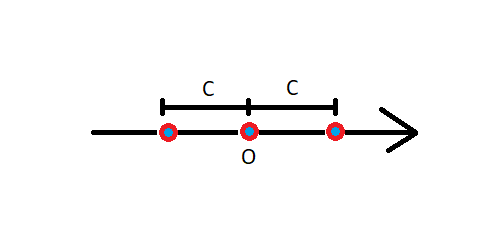简述

$\sum_{n = 1}^\infty a_n(x - c)^n$

$\sum_{n = 1}^\infty a_n(x - c)^n = a_1(x - c) + a_2(x - c)^2 + a_3(x - c)^3 + \cdots + a_n(x - c)^n$

幂级数的研究

幂级数的研究方向

• 幂级数的收敛半径和收敛域
• 幂级数的和函数
• 函数的幂级数展开
其中幂级数的和函数需要用到等比级数中的知识（如其求和公式 $S_n = \frac {\rm 首项}{1 - {\rm 公比}}$

幂级数的收敛半径和收敛域的定义幂级数的和函数定义

• 幂级数在公共收敛区域内可以逐项加减
• 幂级数若在区间 $(-R,R)$ 内有各阶导数，则可以逐项取导，其结果收敛半径不变（两端点敛散性可能改变）
• 幂级数若在区间 $(-R,R)$ 内可积，则可以逐项积分，其结果收敛半径不变（两端点敛散性可能改变）

求和函数的方法

• 思考如何将原幂级数化为等比级数形式
• 利用等比级数进行求和
• 将积分或求导的结果还原为 $s(x)$

函数的幂级数展开

$f(x) = \sum_{n=0}^\infty \frac {f^{(n)}(x_0)} {n!} (x-x_0)^n +\frac {f^{(n+1)}(\xi)} {(n+1)!} (x-x_0)^{(n+1)}$

$f(x) = \sum_{n=0}^\infty \frac {f^{(n)}(x_0)} {n!} (x-x_0)^n$

$f(x) = \sum_{n=0}^\infty \frac {f^{(n)}(0)} {n!} (x)^n$

函数的幂级数展开方法

• 求出原函数 $f(x)$$n$ 阶导数 $f^{(n)}(x)$
• 代入 $x_0=0$ 进行计算
• 将第二步计算的结果一项项代入麦克劳林标准式
• 整理并写出通项
• 找出级数收敛域
• 证明 $\lim_{(n\to\infty)}Rn(x)=0$
注意，在证明 $\lim_{(n\to\infty)}Rn(x)=0$ 时我们会用到夹挤定理，将 $\lim_{(n\to\infty)}Rn(x)=0$ 的值取绝对值并扩大并证明扩大后的值趋近于 0。要证明扩大后的值趋近于 0，我们可以将该项直接作为通项放入一个级数当中并采用比值审敛法。如果该级数收敛，那么根据级数的定义，该级数通项趋近于 0。由此我们可以证明到 $\lim_{(n\to\infty)}Rn(x)=0$，所以该麦克劳林级数收敛于原函数。

$Rn(x)=\frac {e^\xi} {(n+1)!}x^{n+1}$

$|Rn(x)|=\frac {e^\xi} {(n+1)!}|x|^{n+1}\le\frac {e^{|\xi|}} {(n+1)!}|x|^{n+1}

$\sum_{n=1}^\infty\frac {|x|^{n+1}} {(n+1)!}$

End - of - File